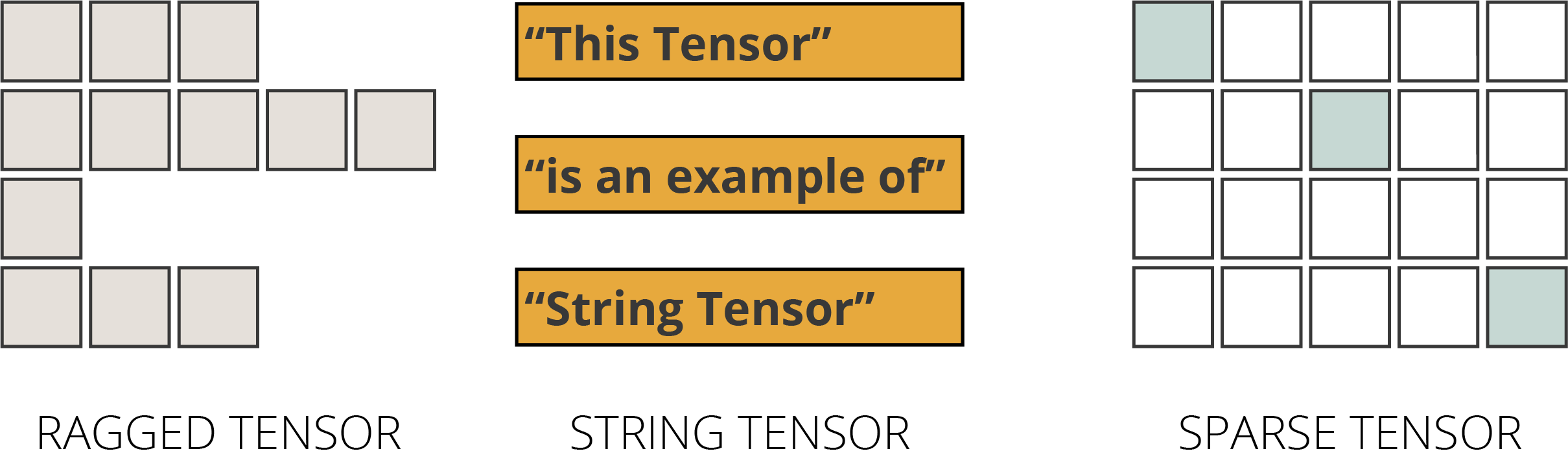# 5个简单的步骤掌握Tensorflow的Tensor• 第一步：张量的定义→什么是张量？
• 第二步：创建张量→创建张量对象的函数
• 第三步：张量对象的特征
• 第四步：张量操作→索引、基本张量操作、形状操作、广播
• 第五步：特殊张量

### 张量的定义：什么是张量import tensorflow as tf
import numpy as np

### 张量的创建：创建张量对象

# 你可以用tf.constant函数创建tf.Tensor对象:
x = tf.constant([[1, 2, 3, 4 ,5]])
# 你可以用tf.ones函数创建tf.Tensor对象:
y = tf.ones((1,5))
# 你可以用tf.zeros函数创建tf.Tensor对象:
z = tf.zeros((1,5))
# 你可以用tf.range函数创建tf.Tensor对象:
q = tf.range(start=1, limit=6, delta=1)

print(x)
print(y)
print(z)
print(q)
输出：

tf.Tensor([[1 2 3 4 5]], shape=(1, 5), dtype=int32)
tf.Tensor([[1. 1. 1. 1. 1.]], shape=(1, 5), dtype=float32)
tf.Tensor([[0. 0. 0. 0. 0.]], shape=(1, 5), dtype=float32)
tf.Tensor([1 2 3 4 5], shape=(5,), dtype=int32)

### 张量对象的特征

tf.Tensor创建对象，它们有几个特征。首先，他们有维度数量。其次，它们有一个形状，一个由维度的长度组成的列表。所有张量都有一个大小，即张量中元素的总数。最后，它们的元素都被记录在一个统一的数据类型（datatype）中。让我们仔细看看这些特征。

#### 维度

• Rank-0（标量）张量：包含单个值且没有轴的张量（0维）；
• Rank-1张量：包含单轴（一维）值列表的张量；
• Rank-2张量：包含2个轴（2维）的张量；以及
• Rank-N张量：包含N轴的张量（三维）。three_level_nested_list = [[[0, 1, 2],
[3, 4, 5]],
[[6, 7, 8],
[9, 10, 11]] ]
rank_3_tensor = tf.constant(three_level_nested_list)
print(rank_3_tensor)
Output:
tf.Tensor( [[[ 0  1  2]
[ 3  4  5]]

[[ 6  7  8]
[ 9 10 11]]],
shape=(2, 2, 3), dtype=int32)

tensor_ndim = rank_3_tensor.ndim
print("The number of dimensions in our Tensor object is", tensor_ndim)
Output:
The number of dimensions in our Tensor object is 3

#### 形状

tensor_shape = rank_3_tensor.shape
print("The shape of our Tensor object is", tensor_shape)
Output:
The shape of our Tensor object is (2, 2, 3)

#### 大小

tensor_size = tf.size(rank_3_tensor).numpy()
print("The size of our Tensor object is", tensor_size)
Output:
The size of our Tensor object is 12

#### 数据类型

tensor_dtype = rank_3_tensor.dtype
print("The data type selected for this Tensor object is", tensor_dtype)
Output:
The data type selected for this Tensor object is <dtype: 'int32'>

### 张量运算

#### 索引

TensorFlow还遵循标准的Python索引规则，这类似于列表索引或NumPy数组索引。

1. 索引从零（0）开始。
2. 负索引（“-n”）值表示从末尾向后计数。
3. 冒号（“：”）用于切片：开始：停止：步骤。
4. 逗号（“，”）用于达到更深层次。

single_level_nested_list = [0, 1, 2, 3, 4, 5, 6, 7, 8, 9, 10, 11]
rank_1_tensor = tf.constant(single_level_nested_list)
print(rank_1_tensor)
Output:
tf.Tensor([ 0  1  2  3  4  5  6  7  8  9 10 11],
shape=(12,), dtype=int32)

# 规则1，索引从0开始
print("First element is:",
rank_1_tensor.numpy())

# 规则2，负索引
print("Last element is:",
rank_1_tensor[-1].numpy())

# 规则3，切片
print("Elements in between the 1st and the last are:",
rank_1_tensor[1:-1].numpy())
Output:
First element is: 0
Last element is: 11
Elements in between the 1st and the last are: [ 1  2  3  4  5  6  7  8  9 10]

two_level_nested_list = [ [0, 1, 2, 3, 4, 5], [6, 7, 8, 9, 10, 11] ]
rank_2_tensor = tf.constant(two_level_nested_list)
print(rank_2_tensor)
Output:
tf.Tensor( [[ 0  1  2  3  4  5]
[ 6  7  8  9 10 11]], shape=(2, 6), dtype=int32)

print("The 1st element of the first level is:",
rank_2_tensor.numpy())

print("The 2nd element of the first level is:",
rank_2_tensor.numpy())

# 规则4, 逗号代表进入更深层
print("The 1st element of the second level is:",
rank_2_tensor[0, 0].numpy())

print("The 3rd element of the second level is:",
rank_2_tensor[0, 2].numpy())
Output:
The first element of the first level is: [0 1 2 3 4 5]
The second element of the first level is: [ 6  7  8  9 10 11]
The first element of the second level is: 0
The third element of the second level is: 2

### 张量基本运算

1. 加法
2. 元素乘法
3. 矩阵乘法
4. 求最大值或最小值
5. 找到Max元素的索引
6. 计算Softmax值

a = tf.constant([[2, 4],
[6, 8]], dtype=tf.float32)
b = tf.constant([[1, 3],
[5, 7]], dtype=tf.float32)

# 我们可以使用' tf.add() '函数并将张量作为参数传递。
print(add_tensors)
Output:
tf.Tensor( [[ 3.  7.]
[11. 15.]], shape=(2, 2), dtype=float32)

# 我们可以使用' tf.multiply() '函数并将张量作为参数传递。
multiply_tensors = tf.multiply(a,b)
print(multiply_tensors)
Output:
tf.Tensor( [[ 2. 12.]
[30. 56.]], shape=(2, 2), dtype=float32)

# 我们可以使用' tf.matmul() '函数并将张量作为参数传递。
matmul_tensors = tf.matmul(a,b)
print(matmul_tensors)
Output:
tf.Tensor( [[ 2. 12.]
[30. 56.]], shape=(2, 2), dtype=float32)

# 使用' tf.reduce_max() '和' tf.reduce_min() '函数可以找到最大值或最小值
print("The Max value of the tensor object b is:",
tf.reduce_max(b).numpy())

# 使用' tf.argmax() '函数可以找到最大元素的索引
print("The index position of the max element of the tensor object b is:",
tf.argmax(b).numpy())

# 使用 tf.nn.softmax'函数计算softmax
print("The softmax computation result of the tensor object b is:",
tf.nn.softmax(b).numpy())
Output:
The Max value of the tensor object b is: 1.0
The index position of the Max of the tensor object b is: [1 1]
The softmax computation result of the tensor object b is: [[0.11920291 0.880797  ]  [0.11920291 0.880797  ]]

#### 操纵形状

# 我们的初始张量
a = tf.constant([[1, 2, 3, 4, 5, 6]])
print('The shape of the initial Tensor object is:', a.shape)

b = tf.reshape(a, [6, 1])
print('The shape of the first reshaped Tensor object is:', b.shape)

c = tf.reshape(a, [3, 2])
print('The shape of the second reshaped Tensor object is:', c.shape)

# 如果我们以shape参数传递-1，那么张量就变平坦化。
print('The shape of the flattened Tensor object is:', tf.reshape(a, [-1]))
Output:
The shape of our initial Tensor object is: (1, 6)
The shape of our initial Tensor object is: (6, 1)
The shape of our initial Tensor object is: (3, 2)
The shape of our flattened Tensor object is: tf.Tensor([1 2 3 4 5 6], shape=(6,), dtype=int32)

#### 广播

m = tf.constant()

n = tf.constant([[1,2],[3,4]])

print(tf.multiply(m, n))
Output:
tf.Tensor( [[ 5 10]
[15 20]], shape=(2, 2), dtype=int32)

### 张量的特殊类型

1. 参差不齐的张量
2. 字符串张量
3. 稀疏张量#### 参差不齐的张量

ragged_list = [[1, 2, 3],[4, 5],]

ragged_tensor = tf.ragged.constant(ragged_list)

print(ragged_tensor)
Output:
<tf.RaggedTensor [[1, 2, 3],
[4, 5],
]>

#### 字符串张量

string_tensor = tf.constant(["With this",
"code, I am",
"creating a String Tensor"])

print(string_tensor)
Output:
tf.Tensor([b'With this'
b'code, I am'
b'creating a String Tensor'],
shape=(3,), dtype=string)

#### 稀疏张量

sparse_tensor = tf.sparse.SparseTensor(indices=[[0, 0], [2, 2], [4, 4]],
values=[25, 50, 100],
dense_shape=[5, 5])

# 我们可以把稀疏张量转换成密集张量
print(tf.sparse.to_dense(sparse_tensor))
Output:
tf.Tensor( [[ 25   0   0   0   0]
[  0   0   0   0   0]
[  0   0  50   0   0]
[  0   0   0   0   0]
[  0   0   0   0 100]], shape=(5, 5), dtype=int32)

### 结尾

http://panchuang.net/

sklearn机器学习中文官方文档：
http://sklearn123.com/

http://docs.panchuang.net/